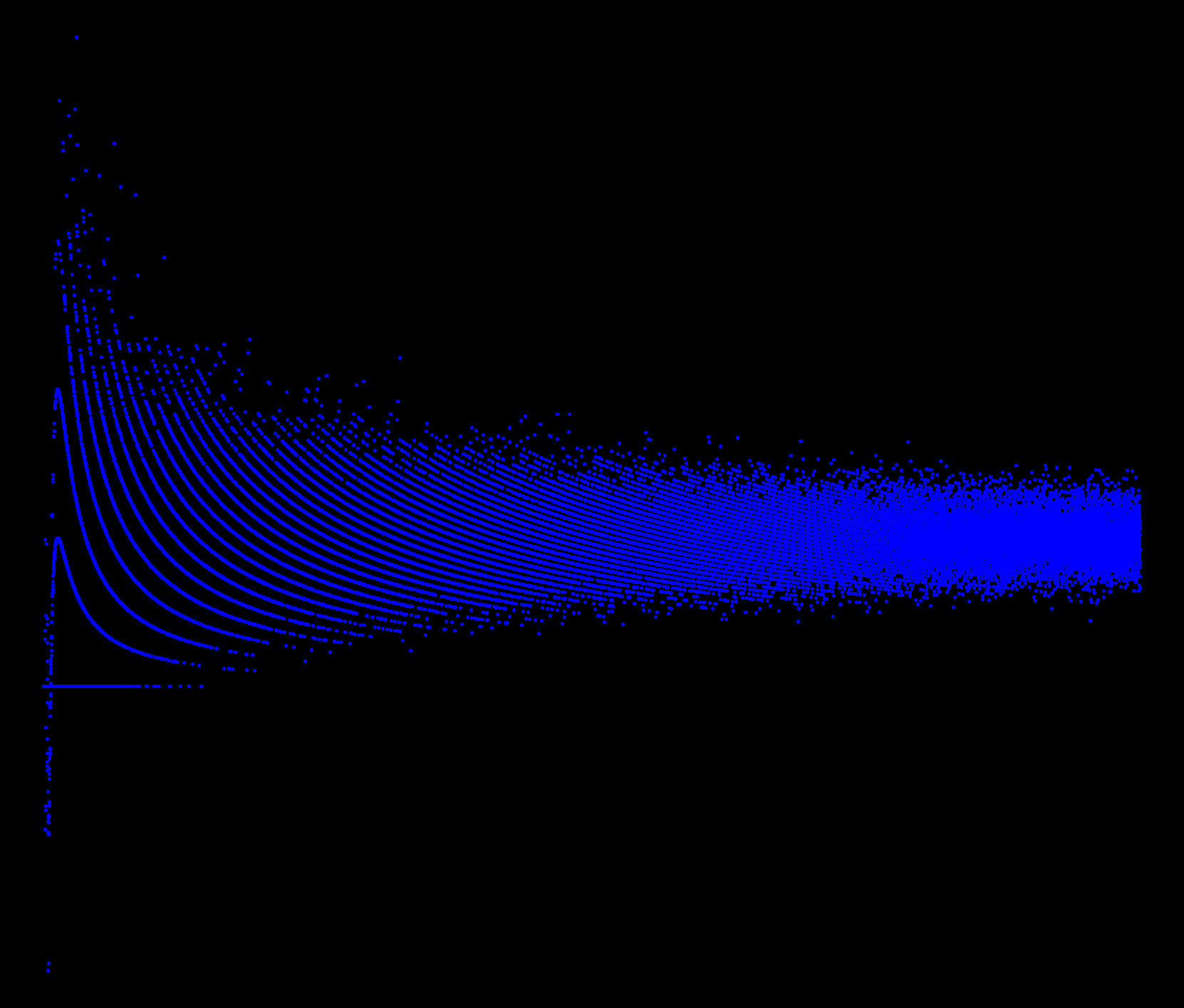## R: More plotting fun with PoissionCoded as follows:

```x = seq(.001,50,.001)
par(bg="black")
par(mar=c(0,0,0,0))
plot(x,sin(1/x)*rpois(length(x),x),pch=20,col="blue")
```

Tags: ,

### One comment

1.Tim Chan

awesome. what program generated this?